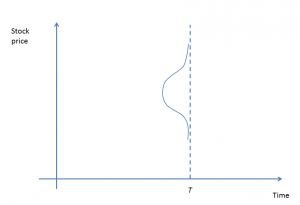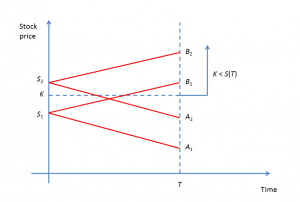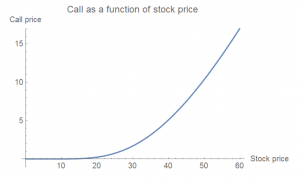20
Feb 18

## Call options and probabilistic intuition - dependence on stock price

The "Why do we want options?" section should be enough to understand that the call price grows when the stock price increases. Here we look at a more technical (linked to probabilities) explanation of that fact.

## Stock price as a stochastic process

The stock price is a stochastic process. That is, it depends on two variables: time$T$ and element of the sample space$\omega$,$S=S(T,\omega)$.Figure 1. Stock price distribution

If we fix time, we obtain a random variable whose density can be visualized as in Figure 1 (the element of the sample space is varying along the vertical line through$T$). The dependence on$\omega$ most of the time is not explicitly mentioned.Figure 2. Stock price

If we fix the element of the sample space, we obtain a function of time, which is a particular path that the stock price may follow. It looks much like a Brownian motion path that I downloaded from Google images, except that the stock price cannot be negative, see Figure 2.

The distribution in Figure 1 looks like normal. Normal distributions are not good for modeling stock prices because stock prices cannot be negative or very large. In our analysis we use triangular distributions, which are isosceles triangles with area equal to one. Without any knowledge about the company and its stock, the best guess that we can make about the mean of the stock price$ES(T)$ at a future time is that it is equal to the stock price now$S(0)$.

Assumption 1. The distribution of$S(T)$ is triangular and it is centered at$S(0)$.Figure 3. Triangular distribution

The result of this assumption is Figure 3, where the triangle ABC describes the density of$S(T)$. We also need the triangle S(0)AB, which describes the area of influence of the price$S(0)$: stock price paths that start at$S(0)$ may end only in the base AB of the triangle. Note that if the price$S(0)$ is low, then the triangle S(0)AB intersects the time line and negative prices will be possible. To avoid complications, we exclude low stock prices.

## Influence of stock price on call priceFigure 4. Call price as a function of stock price

In Figure 4 we compare two initial stock prices:$S_1. Recall that the call price is higher if the likelihood of the event$\{K is higher. For$S_1$ the influence area is the segment$A_1B_1$, which has a smaller intersection with$\{K than the segment$A_2B_2$, which is the influence area of$S_2$. Thus, the call price corresponding to$S_2$ should be higher than the one corresponding to$S_1$.Figure 5. Call as a function of stock price

Figure 5 from Mathematica concludes this post. In that Figure, the strike price is assumed to be $50. The very low call price at stock prices below$20 is due to the fact that it is not very likely for the stock to become in the money if it starts that low.